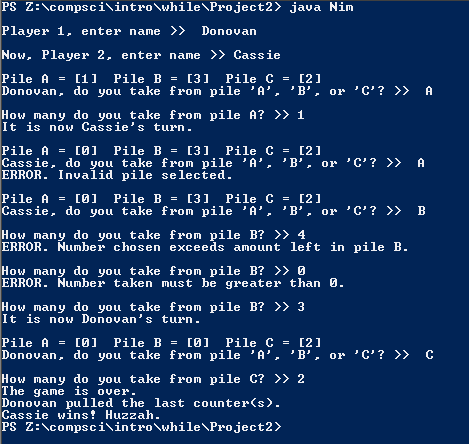# Project 2; Nim

## Code

```    // Donovan Rich
// Period 6
// Nim
// Nim.java
// 2/5/2016

import java.util.Scanner;
import java.util.Random;

public class Nim
{
public static void main( String[] args )
{
Scanner keyboard = new Scanner(System.in);
Random r = new Random();
String selector="", player1, player2;
int p1 = 1+r.nextInt(5), p2 = 1+r.nextInt(5), p3 = 1+r.nextInt(5), turn = 1, nimber = 0;
System.out.print( "\nPlayer 1, enter name >>  " );
player1 = keyboard.next();
System.out.print( "\nNow, Player 2, enter name >> " );
player2 = keyboard.next();

while ( p1+p2+p3 != 0 )
{
while ( turn == 1  )
{
System.out.println( "\nPile A = [" + p1 + "]  Pile B = [" + p2 + "]  Pile C = [" + p3 + "]" );
System.out.print( player1 + ", do you take from pile 'A', 'B', or 'C'? >>  " );
selector = keyboard.next();
if ( ( selector.equals("A") && p1 > 0 ) || ( selector.equals("B") && p2 > 0 )|| ( selector.equals("C") && p3 > 0 ) )
{
do
{
if ( selector.equals("A") )
{
System.out.print( "\nHow many do you take from pile A? >> " );
nimber = keyboard.nextInt();
if ( nimber < 1 )
System.out.println( "ERROR. Number taken must be greater than 0." );
else if ( nimber > p1 )
System.out.println( "ERROR. Number chosen exceeds amount left in pile A." );
else
{
p1 = p1 - nimber;
turn = 2;
System.out.println( "It is now " + player2 + "'s turn." );
}
}
if ( selector.equals("B") )
{
System.out.print( "\nHow many do you take from pile B? >> " );
nimber = keyboard.nextInt();
if ( nimber < 1 )
System.out.println( "ERROR. Number taken must be greater than 0." );
else if ( nimber > p2 )
System.out.println( "ERROR. Number chosen exceeds amount left in pile B." );
else
{
p2 = p2 - nimber;
turn = 2;
System.out.println( "It is now " + player2 + "'s turn." );
}
}
if ( selector.equals("C") )
{
System.out.print( "\nHow many do you take from pile C? >> " );
nimber = keyboard.nextInt();
if ( nimber < 1 )
System.out.println( "ERROR. Number taken must be greater than 0." );
else if ( nimber > p3 )
System.out.println( "ERROR. Number chosen exceeds amount left in pile C." );
else
{
p3 = p3 - nimber;
turn = 2;
if ( p1+p2+p3 != 0 )
System.out.println( "\nIt is now " + player2 + "'s turn." );
}
}
} while ( turn == 1 );
}
else
System.out.println( "ERROR. Invalid pile selected." );

}
while ( turn == 2 && p1+p2+p3 != 0 )
{
System.out.println( "\nPile A = [" + p1 + "]  Pile B = [" + p2 + "]  Pile C = [" + p3 + "]" );
System.out.print( player2 + ", do you take from pile 'A', 'B', or 'C'? >>  " );
selector = keyboard.next();
if ( ( selector.equals("A") && p1 > 0 ) || ( selector.equals("B") && p2 > 0 )|| ( selector.equals("C") && p3 > 0 ) )
{
do
{
if ( selector.equals("A") )
{
System.out.print( "\nHow many do you take from pile A? >> " );
nimber = keyboard.nextInt();
if ( nimber < 1 )
System.out.println( "ERROR. Number taken must be greater than 0." );
else if ( nimber > p1 )
System.out.println( "ERROR. Number chosen exceeds amount left in pile A." );
else
{
p1 = p1 - nimber;
turn = 1;
System.out.println( "It is now " + player1 + "'s turn." );
}
}
if ( selector.equals("B") )
{
System.out.print( "\nHow many do you take from pile B? >> " );
nimber = keyboard.nextInt();
if ( nimber < 1 )
System.out.println( "ERROR. Number taken must be greater than 0." );
else if ( nimber > p2 )
System.out.println( "ERROR. Number chosen exceeds amount left in pile B." );
else
{
p2 = p2 - nimber;
turn = 1;
System.out.println( "It is now " + player1 + "'s turn." );
}
}
if ( selector.equals("C") )
{
System.out.print( "\nHow many do you take from pile C? >> " );
nimber = keyboard.nextInt();
if ( nimber < 1 )
System.out.println( "ERROR. Number taken must be greater than 0." );
else if ( nimber > p3 )
System.out.println( "ERROR. Number chosen exceeds amount left in pile C." );
else
{
p3 = p3 - nimber;
turn = 1;
if ( p1+p2+p3 != 0 )
System.out.println( "\nIt is now " + player1 + "'s turn." ); //IT IS NOW POOP'S TURN
}
}
} while ( turn == 2 );
}
else
System.out.println( "ERROR. Invalid pile selected." );

}
}
System.out.println( "The game is over." );
if ( turn == 1 )
{
System.out.println( player2 + " pulled the last counter(s)." );
System.out.println( player1 + " wins! Huzzah. " );
}
else if ( turn == 2 )
{
System.out.println( player1 + " pulled the last counter(s)." );
System.out.println( player2 + " wins! Huzzah. " );
}

}
}
```

### Picture of the output